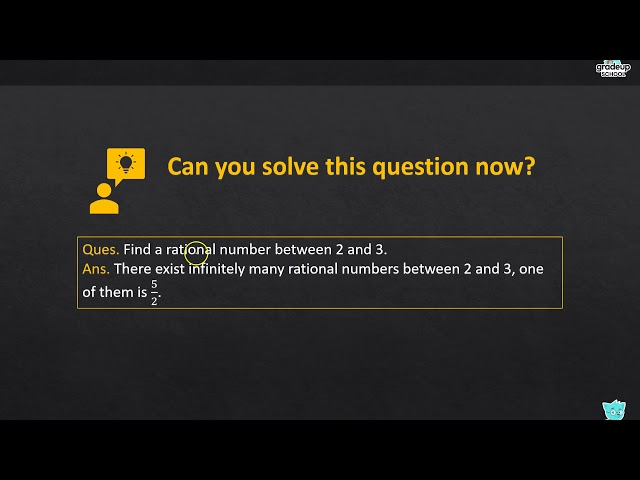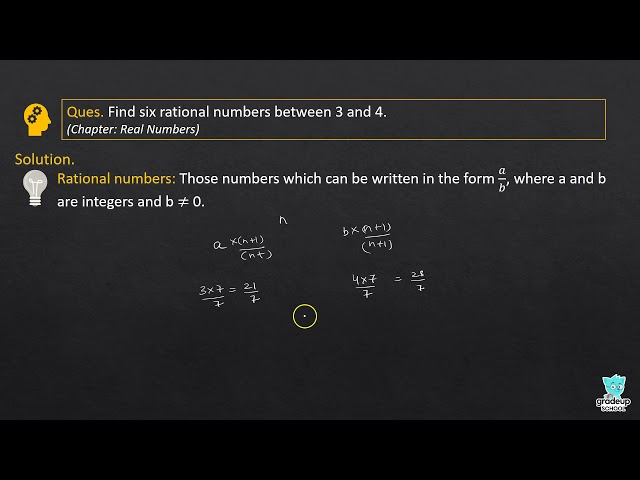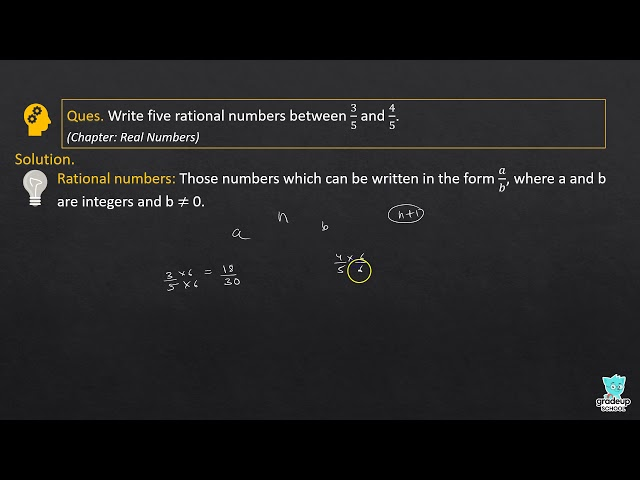# NCERT Solutions for Class 9 Maths Chapter 1 - Number System

Share

NCERT Solutions For Class 9 Maths Chapter 1 – Number System have been made available here for free of cost. These Solutions for Number Systems have been prepared flawlessly by the expert teachers of the Maths. Also, the Solutions are based on the present syllabus of the CBSE which all the more enhances their value.

The Chapter 1 – Number Systems comprise of important topics such as Irrational Numbers, Real Numbers and their Decimal Expansions, Operations on Real Numbers as well as Laws of Exponents on Real Numbers. The Chapter forms an integral part of the 9th Class Maths syllabus and therefore requires to be prepared thoroughly. By using our NCERT Solutions for Maths Chapter 1, you can develop a proper understanding of the important topics and clear your doubts. This will enhance the level of your subject preparation and would enable you to perform better in the exam.

## NCERT Solutions for Class 9 Maths Chapter 1 - Number System

Exercise 1.1
• Exercise 1.1
• Exercise 1.2
• Exercise 1.3
• Exercise 1.4
• Exercise 1.5
• Exercise 1.6
Class 9th|NCERT - MathematicsChapter 1 - Number SystemNCERT - MathematicsClass 9th , NCERT
 Chapter 1 - Number System Chapter 2 - Polynomials Chapter 3 - Coordinate Geometry Chapter 4 - Linear Equations in two Variables Chapter 5 - Introduction to Euclid's Geometry Chapter 6 - Lines and Angles Chapter 7 - Triangles Chapter 8 - Quadrilaterals Chapter 9 - Areas of Parallelograms and Triangles Chapter 10 - Circles Chapter 11 - Constructions Chapter 12 - Heron's Formula Chapter 13 - Surface Areas and Volumes Chapter 14 - Statistics Chapter 15 - Probability